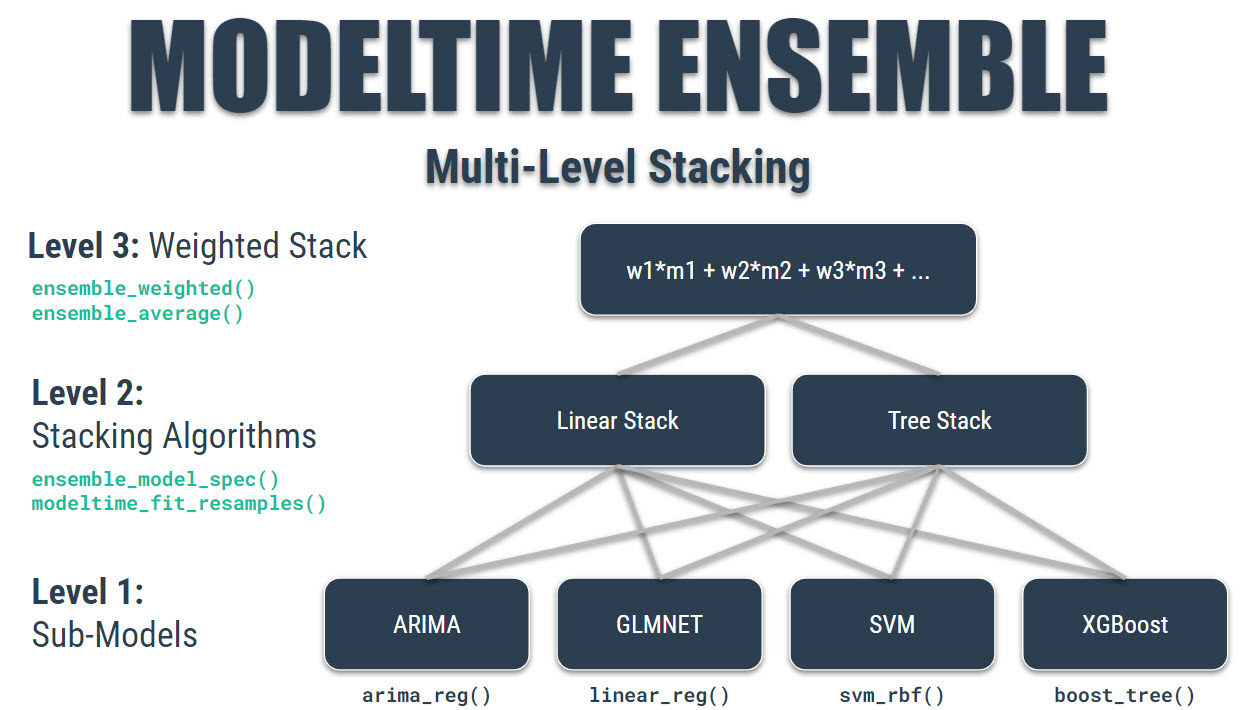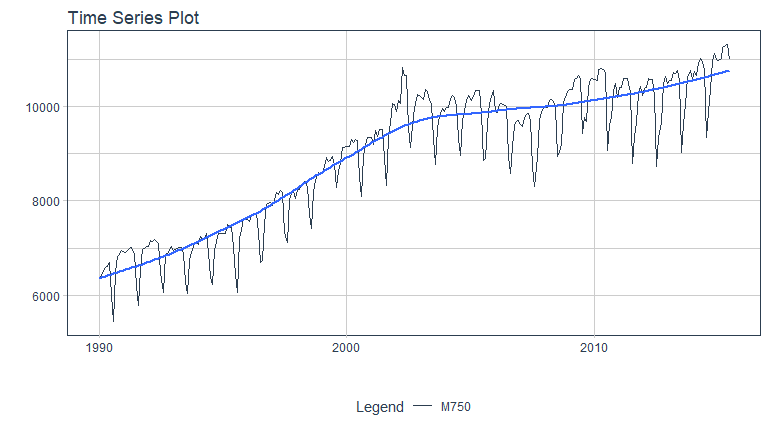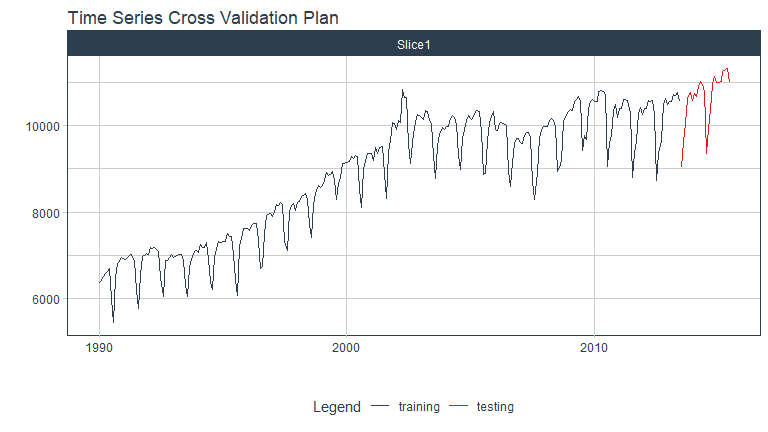# Getting Started with Modeltime Ensemble

Ensemble Algorithms for Time Series Forecasting with Modeltime

A modeltime extension that that implements ensemble forecasting methods including model averaging, weighted averaging, and stacking. Let’s go through a guided tour to kick the tires on modeltime.ensemble.# Time Series Ensemble Forecasting Example

We’ll perform the simplest type of forecasting: Using a simple average of the forecasted models.

Note that modeltime.ensemble has capabilities for more sophisticated model ensembling using:

• Weighted Averaging
• Stacking using an Elastic Net regression model (meta-learning)

## Libraries

Load libraries to complete this short tutorial.

# Time Series ML
library(tidymodels)
library(modeltime)
library(modeltime.ensemble)

# Core
library(tidyverse)
library(timetk)

interactive <- FALSE

## Collect the Data

We’ll use the m750 dataset that comes with modeltime.ensemble. We can visualize the dataset.

m750 %>%
plot_time_series(date, value, .color_var = id, .interactive = interactive)# Perform Train / Test Splitting

We’ll split into a training and testing set.

splits <- time_series_split(m750, assess = "2 years", cumulative = TRUE)

splits %>%
tk_time_series_cv_plan() %>%
plot_time_series_cv_plan(date, value, .interactive = interactive)# Modeling

Once the data has been collected, we can move into modeling.

## Recipe

We’ll create a Feature Engineering Recipe that can be applied to the data to create features that machine learning models can key in on. This will be most useful for the Elastic Net (Model 3).

recipe_spec <- recipe(value ~ date, training(splits)) %>%
step_timeseries_signature(date) %>%
step_rm(matches("(.iso$)|(.xts$)")) %>%
step_normalize(matches("(index.num$)|(_year$)")) %>%
step_dummy(all_nominal()) %>%
step_fourier(date, K = 1, period = 12)

recipe_spec %>% prep() %>% juice()
#> # A tibble: 282 x 42
#>    date       value date_index.num date_year date_half date_quarter date_month
#>    <date>     <dbl>          <dbl>     <dbl>     <int>        <int>      <int>
#>  1 1990-01-01  6370          -1.72     -1.66         1            1          1
#>  2 1990-02-01  6430          -1.71     -1.66         1            1          2
#>  3 1990-03-01  6520          -1.70     -1.66         1            1          3
#>  4 1990-04-01  6580          -1.69     -1.66         1            2          4
#>  5 1990-05-01  6620          -1.67     -1.66         1            2          5
#>  6 1990-06-01  6690          -1.66     -1.66         1            2          6
#>  7 1990-07-01  6000          -1.65     -1.66         2            3          7
#>  8 1990-08-01  5450          -1.64     -1.66         2            3          8
#>  9 1990-09-01  6480          -1.62     -1.66         2            3          9
#> 10 1990-10-01  6820          -1.61     -1.66         2            4         10
#> # ... with 272 more rows, and 35 more variables: date_day <int>,
#> #   date_hour <int>, date_minute <int>, date_second <int>, date_hour12 <int>,
#> #   date_am.pm <int>, date_wday <int>, date_mday <int>, date_qday <int>,
#> #   date_yday <int>, date_mweek <int>, date_week <int>, date_week2 <int>,
#> #   date_week3 <int>, date_week4 <int>, date_mday7 <int>,
#> #   date_month.lbl_01 <dbl>, date_month.lbl_02 <dbl>, date_month.lbl_03 <dbl>,
#> #   date_month.lbl_04 <dbl>, date_month.lbl_05 <dbl>, ...

## Model 1 - Auto ARIMA

First, we’ll make an ARIMA model using Auto ARIMA.

model_spec_arima <- arima_reg() %>%
set_engine("auto_arima")

wflw_fit_arima <- workflow() %>%
fit(training(splits))

## Model 2 - Prophet

Next, we’ll make a Prophet Model.

model_spec_prophet <- prophet_reg() %>%
set_engine("prophet")

wflw_fit_prophet <- workflow() %>%
fit(training(splits))

## Model 3 - Elastic Net

Third, we’ll make an Elastic Net Model using glmnet.

model_spec_glmnet <- linear_reg(
mixture = 0.9,
penalty = 4.36e-6
) %>%
set_engine("glmnet")

wflw_fit_glmnet <- workflow() %>%
fit(training(splits))